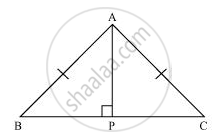# ABC is an isosceles triangle with AB = AC. Drawn AP ⊥ BC to show that ∠B = ∠C. - Mathematics

ABC is an isosceles triangle with AB = AC. Drawn AP ⊥ BC to show that ∠B = ∠C.

#### SolutionIn ΔAPB and ΔAPC,

∠APB = ∠APC (Each 90º)

AB =AC (Given)

AP = AP (Common)

∴ ΔAPB ≅ ΔAPC (Using RHS congruence rule)

⇒ ∠B = ∠C (By using CPCT)

Concept: Some More Criteria for Congruence of Triangles
Is there an error in this question or solution?
Chapter 7: Triangles - Exercise 7.3 [Page 128]

#### APPEARS IN

NCERT Class 9 Maths
Chapter 7 Triangles
Exercise 7.3 | Q 5 | Page 128

Share